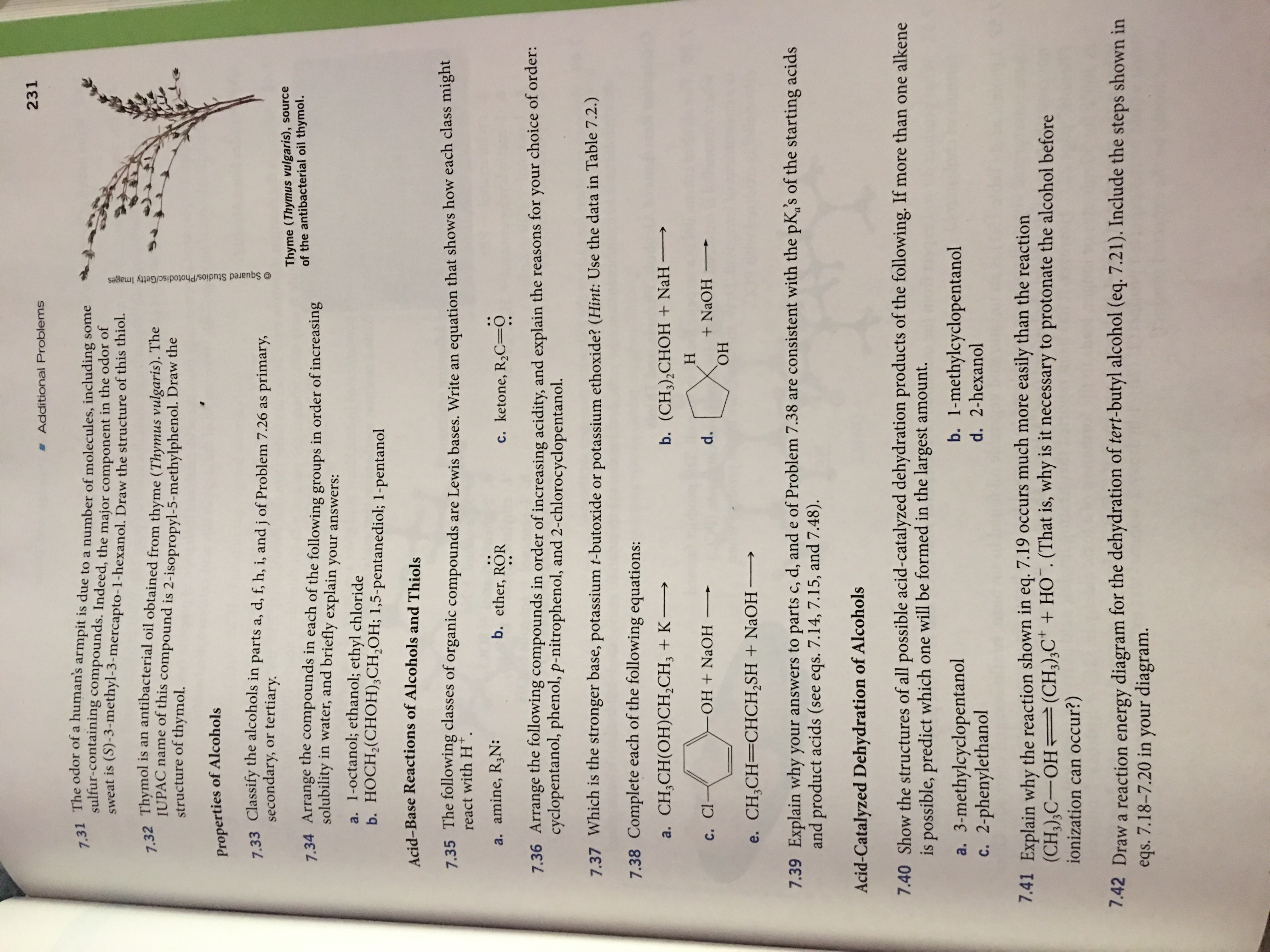# 231 Additional Problems 7.31 The odor of a human's armpit is due to a number of molecules, including some sulfur-containing compounds. Indeed, the major component in the odor of sweat is (S)-3-methyl-3-mercapto-1-hexanol. Draw the structure of this thiol. 7.32 Thymol is an antibacterial oil obtained from thyme (Thymus vulgaris). The IUPAC name of this compound is 2-isopropyl-5-methylphenol. Draw the structure of thymol. Properties of Alcoh ols 7.33 Classify the alcohols in parts a, d, f, h, i, and j of Problem 7.26 as primary, secondary, or tertiary Thyme (Thymus vulgaris), source of the antibacterial oil thymol. 7.34 Arrange the compounds in each of the following groups in order of increasing solubility in water, and briefly explain your answers: a. 1-octanol; ethanol; ethyl chloride b. HOCH2(CHOH)3CH2OH; 1,5-pentanediol; 1-pentanol Acid-Base Reactions of Alcohols and Thiols 7.35 The following classes of organic compounds are Lewis bases. Write an equation that shows how each class might react with H+ a. amine, R3N: b. ether, ROR c. ketone, R2C=0 choice of order: 7.36 Arrange the following compounds in order of increasing acidity, and explain the reasons for cyclopentanol, phenol, p-nitrophenol, and 2-chlorocyclopentanol. your 7.37 Which is the stronger base, potassium t-butoxide or potassium ethoxide? (Hint: Use the data in Table 7.2.) 7.38 Complete each of the following equations: a. CH,CH(ОН)CH,CH, + K — b. (CH),СHОН + NaH — Н +NaOH ОН d. OH NaOH С. СI- e. CH2CH=CHCH2SH + NaOH> 7.39 Explain why your answers to parts c, d, and e of Problem 7.38 are consistent with the pKgs of the starting acids and product acids (see eqs. 7.14, 7.15, and 7.48) Acid-Catalyzed Dehydration of Alcohols 7.40 Show the structures of all possible acid-catalyzed dehydration products of the following. If more than one alkene is possible, predict which one will be formed in the largest amount. b. 1-methylcyclopentanol d. 2-hexanol a. 3-methylcyclopentanol c. 2-phenylethanol 7.41 Explain why the reaction shown in eq. 7.19 occurs much more easily than the reaction (CH3)3C-OH (CH),C + HO. (That is, why is it necessary to protonate the alcohol before ionization can occur?) 7.42 Draw a reaction energy diagram for the dehydration of tert-butyl alcohol (eq. 7.21). Include the steps shown in eqs. 7.18-7.20 in your diagram. Squared Studios/Photodisc/Getty Images

Question

7.40 a, b, c

*Can you write each step put w/an explanation...please and thank youhelp_outlineImage Transcriptionclose231 Additional Problems 7.31 The odor of a human's armpit is due to a number of molecules, including some sulfur-containing compounds. Indeed, the major component in the odor of sweat is (S)-3-methyl-3-mercapto-1-hexanol. Draw the structure of this thiol. 7.32 Thymol is an antibacterial oil obtained from thyme (Thymus vulgaris). The IUPAC name of this compound is 2-isopropyl-5-methylphenol. Draw the structure of thymol. Properties of Alcoh ols 7.33 Classify the alcohols in parts a, d, f, h, i, and j of Problem 7.26 as primary, secondary, or tertiary Thyme (Thymus vulgaris), source of the antibacterial oil thymol. 7.34 Arrange the compounds in each of the following groups in order of increasing solubility in water, and briefly explain your answers: a. 1-octanol; ethanol; ethyl chloride b. HOCH2(CHOH)3CH2OH; 1,5-pentanediol; 1-pentanol Acid-Base Reactions of Alcohols and Thiols 7.35 The following classes of organic compounds are Lewis bases. Write an equation that shows how each class might react with H+ a. amine, R3N: b. ether, ROR c. ketone, R2C=0 choice of order: 7.36 Arrange the following compounds in order of increasing acidity, and explain the reasons for cyclopentanol, phenol, p-nitrophenol, and 2-chlorocyclopentanol. your 7.37 Which is the stronger base, potassium t-butoxide or potassium ethoxide? (Hint: Use the data in Table 7.2.) 7.38 Complete each of the following equations: a. CH,CH(ОН)CH,CH, + K — b. (CH),СHОН + NaH — Н +NaOH ОН d. OH NaOH С. СI- e. CH2CH=CHCH2SH + NaOH> 7.39 Explain why your answers to parts c, d, and e of Problem 7.38 are consistent with the pKgs of the starting acids and product acids (see eqs. 7.14, 7.15, and 7.48) Acid-Catalyzed Dehydration of Alcohols 7.40 Show the structures of all possible acid-catalyzed dehydration products of the following. If more than one alkene is possible, predict which one will be formed in the largest amount. b. 1-methylcyclopentanol d. 2-hexanol a. 3-methylcyclopentanol c. 2-phenylethanol 7.41 Explain why the reaction shown in eq. 7.19 occurs much more easily than the reaction (CH3)3C-OH (CH),C + HO. (That is, why is it necessary to protonate the alcohol before ionization can occur?) 7.42 Draw a reaction energy diagram for the dehydration of tert-butyl alcohol (eq. 7.21). Include the steps shown in eqs. 7.18-7.20 in your diagram. Squared Studios/Photodisc/Getty Images fullscreen

### Want to see this answer and more?

Experts are waiting 24/7 to provide step-by-step solutions in as fast as 30 minutes!*

*Response times may vary by subject and question complexity. Median response time is 34 minutes for paid subscribers and may be longer for promotional offers.
Tagged in
Science
Chemistry

### Organic Chemistry